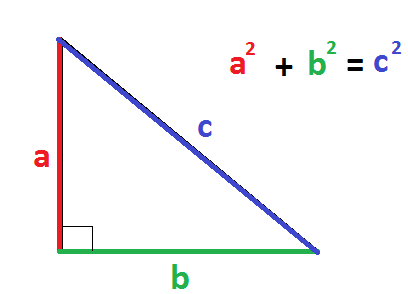# How do you find the missing lengths of the sides of a right triangle given the legs 4 and the hypotenuse 6?

Apr 5, 2018

The missing side length of the right triangle is $\sqrt{20}$ or about $4.47$.

#### Explanation:

When we talk about the side lengths of a right triangle, we typically use the Pythagorean Theorem, as shown here:(study.com)

We are the length of the a leg of the triangle ($4$) and the hypotenuse ($6$).

So we can plug in these values into the equation and solve for the other side length. Let's say that the given side length is $a$:
${\left(4\right)}^{2} + {b}^{2} = {\left(6\right)}^{2}$

And now we simplify:
$16 + {b}^{2} = 36$

Subtract both sides by $16$:
${b}^{2} = 20$

$b = \sqrt{20}$ or about $4.47$